# 02 - Hyperparameter tuning with random search

library(reservoirnet)
library(dplyr)
library(ggplot2)

# Goal

This vignette aims to find the best hyperparameters using randomsearch strategy. We will use the same data as in the vignette 01 basic usage and learn how to find the best set of hyperparameters.

# Get the data

We first load the data :

data("dfCovid")

dist_forecast = 14

traintest_date = as.Date("2022-01-01")

Then we smooth the data to avoid huge variability of RT-PCR :

dfOutcome <- dfCovid %>%
# outcome at 14 days
mutate(outcome = lead(x = hosp, n = dist_forecast),
outcomeDate = date + dist_forecast) %>%
# rolling average for iptcc and positive_pcr
mutate_at(.vars = c("Positive", "Tested"),
.funs = function(x) slider::slide_dbl(.x = x,
.before = 6,
.f = mean))

Now that we have our data ready, we can plot those :

dfOutcome %>%
tidyr::pivot_longer(cols = c("hosp", "Positive", "Tested")) %>%
ggplot2::ggplot(mapping = aes(x = date, y = value)) +
geom_line() +
facet_grid(name ~ ., scales = "free_y") +
theme_bw() +
geom_vline(mapping = aes(color = "train-test sets", xintercept = traintest_date)) +
labs(color = "") +
theme(legend.position = "bottom")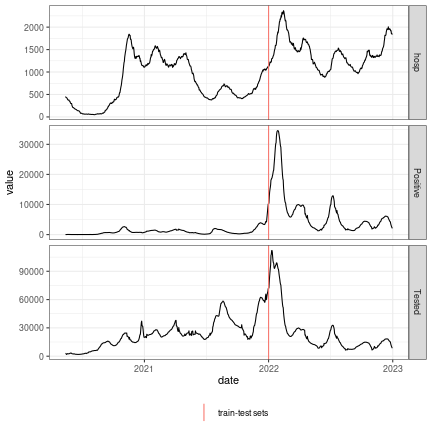Hospitalisations, IPTCC and positive PCR of Bordeaux University Hospital.

# Chose the hyperparameters using random search (setting)

In order to chose the best set of hyperparameters (leaking rate, input scaling, spectral radius and ridge penalty) on the train set we are going to use an accumulate forward procedure. Basically, during the year 2021, we are going to train the model each 6 months and evaluate the forecast on the next 3 months. This procedure will be repeated several times with different sets of hyperparameters each time.

## Preparation

First we set the periods of training and evaluation :

# dates of training
vec_train_sets <- as.Date(c("2021-01-01", "2021-06-01"))
# dates of evaluation
vec_test_sets_start <- vec_train_sets+dist_forecast
vec_test_sets_end <- c(vec_test_sets_start[2:length(vec_test_sets_start)], traintest_date-1)
# get everything in a table
dfaccumulateForward = data.frame(train_date = vec_train_sets,
test_start = vec_test_sets_start,
test_end = vec_test_sets_end)

Then we set the objective functions computing the mse for the chosen of hyperparameters :

fct_objective <- function(ridge,
leaking_rate,
input_scaling,
dfaccumulateForward){
##### reservoir architecture
# set reservoir
reservoir <- reservoirnet::createNode(nodeType = "Reservoir",
units = 500,
lr = 0.7,
sr = 1,
input_scaling = 1)
readout <- reservoirnet::createNode(nodeType = "Ridge", ridge = 0.1)
# connect them

##### evaluate model
dfPredictions <- apply(dfaccumulateForward,
MARGIN = 1,
FUN = function(row_accumulate_forward){
fct_performance_period(model = model,
dfOutcome = dfOutcome,
train_date = as.Date(row_accumulate_forward["train_date"]),
test_start = as.Date(row_accumulate_forward["test_start"]),
test_end = as.Date(row_accumulate_forward["test_end"]))
}) %>%
bind_rows()

##### get performance
mse = dfPredictions %>%
mutate(squared_error = (pred-outcome)^2) %>%
pull(squared_error) %>%
mean(.)

return(mse)
}
fct_performance_period <- function(model,
train_date,
test_start,
test_end,
dfOutcome){
##### train and test set
# train set
yTrain <- dfOutcome %>% filter(outcomeDate <= train_date) %>% select(outcome)
xTrain <- dfOutcome %>% filter(outcomeDate <= train_date) %>% select(hosp, Positive, Tested)
# test set
xTest <- dfOutcome %>% filter(outcomeDate <= test_end) %>% select(hosp, Positive, Tested)
yTest <- dfOutcome %>%
filter(outcomeDate <= test_end) %>%
select(outcomeDate, outcome) %>%
mutate(eval_period = outcomeDate >= test_start)

##### preprocessing of the data
# standardise based on training set values
ls_fct_stand <- apply(xTrain,
MARGIN = 2,
FUN = function(x) function(feature) return(feature/(max(x))))
xTrainstand <- xTrain
xTeststand <- xTest
lapply(X = names(ls_fct_stand),
FUN = function(x){
xTrainstand[,x] <<- ls_fct_stand[[x]](feature = xTrain[,x])
xTeststand[,x] <<- ls_fct_stand[[x]](feature = xTest[,x])
return()
})
# convert to array
lsdf <- lapply(list(yTrain = yTrain,
xTrain = xTrainstand,
xTest = xTeststand),
function(x) as.array(as.matrix(x)))

##### fit reservoir on train set
fit <- reservoirnet::reservoirR_fit(node = model,
X = lsdf$xTrain, Y = lsdf$yTrain,
warmup = 30,
reset = TRUE)
##### predict with the reservoir
vec_pred <- reservoirnet::predict_seq(node = fit$fit, X = lsdf$xTest,
reset = TRUE)
dfPredictions <- yTest %>%
mutate(pred = vec_pred) %>%
filter(eval_period) %>%
select(outcomeDate, outcome, pred)

return(dfPredictions)
}

## Generate hyperparameters

To generate the hyperparameters we use log-uniform generations. This is easily done using the rloguniform and random_search_hyperparam functions. For instance we have :

random_search_hyperparam(
n = 50,
ls_fct = list(
ridge = function(n)
rloguniform(n = n, min = 1e-10, max = 1e-1),
input_scaling = function(n)
1,
rloguniform(n = n, min = 1e-10, max = 1e5),
leaking_rate = function(n)
0.9
)
) %>%
head()
## # A tibble: 6 × 5
##   search_id         ridge input_scaling spectral_radius leaking_rate
##       <int>         <dbl>         <dbl>           <dbl>        <dbl>
## 1         1 0.0000000245              1         1.46e-3          0.9
## 2         2 0.000000223               1         8.28e+2          0.9
## 3         3 0.0000143                 1         3.73e-4          0.9
## 4         4 0.0149                    1         4.70e-7          0.9
## 5         5 0.00000000653             1         1.15e-9          0.9
## 6         6 0.0122                    1         3.10e-9          0.9

# Chose the hyperparameters using random search (results)

To find the right set of hyperparameters, we will keep the ridge hyperparameter random between 1e-10 and 1e-1 and vary other hyperparameters 2 by 2. For each set of hyperparameters, we will run 2 experiment and take the mean mse of the 2. At each step we will run 30 experiments to reduce computation time.

For a real case, it would be better to increase the number of experiment and of repetition to better explore the hyperparameter space.

## Spectral radius and input scaling

dfHyperparam <- random_search_hyperparam(n = 30,
ls_fct = list(ridge = function(n) rloguniform(n = n, min = 1e-10, max = 1e-1),
input_scaling = function(n) rloguniform(n = n, min = 1e-5, max = 1e2),
spectral_radius = function(n) rloguniform(n = n, min = 1e-5, max = 1e2),
leaking_rate = function(n) 0.7)) %>%
# replicate 2 times
replicate(n = 2, simplify = FALSE) %>%
bind_rows() %>%
tibble::rowid_to_column(var = "search_id_master")

vecMSE <- apply(X = dfHyperparam,
MARGIN = 1,
FUN = function(row_hp){
fct_objective(ridge = row_hp["ridge"],
leaking_rate = row_hp["leaking_rate"],
input_scaling = row_hp["input_scaling"],
dfaccumulateForward = dfaccumulateForward)
})

dfPerf = dfHyperparam %>%
select(search_id, search_id_master) %>%
mutate(mse = vecMSE) %>%
group_by(search_id) %>%
summarise(mse = mean(mse)) %>%
left_join(dfHyperparam %>% select(-search_id_master) %>% distinct(),
by = "search_id")

We can now plot the performance using the adequate functions :

plot_2x2_perf(dfPerf %>% select(perf = mse, spectral_radius, input_scaling),
perf_lab = "MSE", trans = "identity")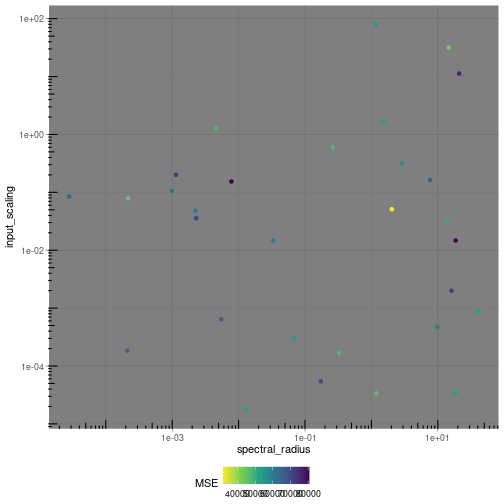plot of chunk plotperf1

plot_marginal_perf(dfPerf %>% select(perf = mse, spectral_radius, input_scaling),
perf_lab = "MSE")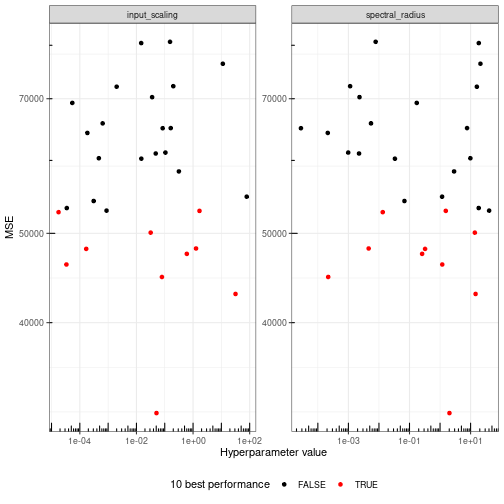plot of chunk plotperf1

There is no clear area of better performance and for a real world application, we should probably increase the number of explored hyperparameter set. Nevertheless, the area where spectral radius and input scaling are equal to 1 seems to have better performance.

We can now move on to tune the leaking rate and the ridge hyperparameters.

## Leaking rate and Ridge

dfHyperparam <- random_search_hyperparam(n = 30,
ls_fct = list(ridge = function(n) rloguniform(n = n, min = 1e-10, max = 1e-1),
input_scaling = function(n) 1,
leaking_rate = function(n) rloguniform(n = n, min = 1e-3, max = 1))) %>%
# replicate 2 times
replicate(n = 2, simplify = FALSE) %>%
bind_rows() %>%
tibble::rowid_to_column(var = "search_id_master")

vecMSE <- apply(X = dfHyperparam,
MARGIN = 1,
FUN = function(row_hp){
fct_objective(ridge = row_hp["ridge"],
leaking_rate = row_hp["leaking_rate"],
input_scaling = row_hp["input_scaling"],
dfaccumulateForward = dfaccumulateForward)
})

dfPerf = dfHyperparam %>%
select(search_id, search_id_master) %>%
mutate(mse = vecMSE) %>%
group_by(search_id) %>%
summarise(mse = mean(mse)) %>%
left_join(dfHyperparam %>% select(-search_id_master) %>% distinct(),
by = "search_id")

We can now plot the performance using the adequate functions :

plot_2x2_perf(dfPerf %>% select(perf = mse, leaking_rate, ridge),
perf_lab = "MSE", trans = "identity")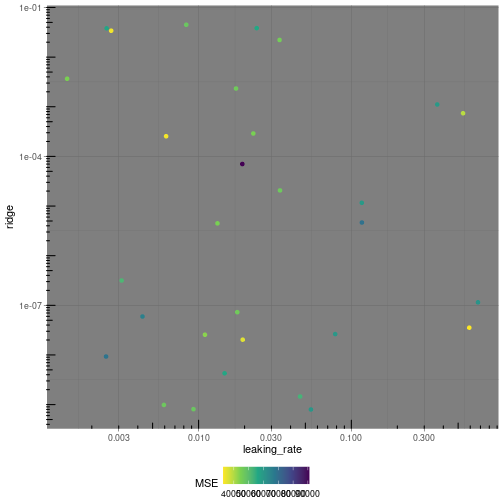plot of chunk plotperf2

plot_marginal_perf(dfPerf %>% select(perf = mse, leaking_rate, ridge),
perf_lab = "MSE")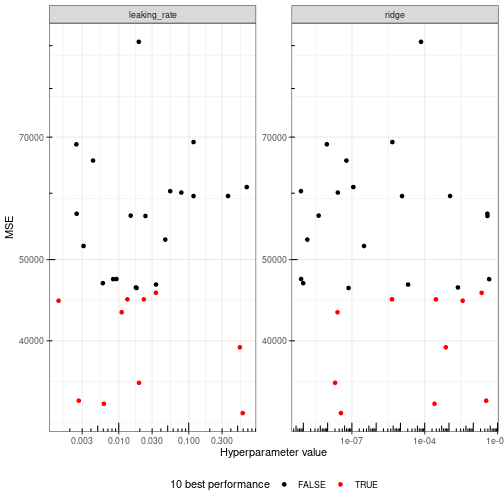plot of chunk plotperf2

Again, for a real world application, we should probably explore a larger set of hyperparameter. Here, we can consider that a leaking rate of 0.5 and a ridge penalty of 1e-3 seem to provide overall best performance. A finer tuning could be done but we stop here for this vignette using random search to find the best set of hyperparameters.